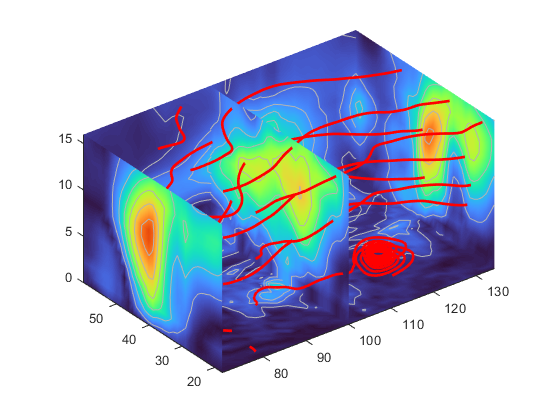使用向量数据显示流线图

风的映射数据

MATLAB® 向量数据集 wind 代表北美地区的气流。本示例结合使用了几种方法：

• 利用流线跟踪风速

• 利用切片平面显示数据的横截面视图

• 利用切片平面上的等高线提高切片平面着色的可见性

1.确定坐标的范围

xmin = min(x(:));
xmax = max(x(:));
ymax = max(y(:));
zmin = min(z(:));

2.添加切片平面以提供视觉环境

wind_speed = sqrt(u.^2 + v.^2 + w.^2);
hsurfaces = slice(x,y,z,wind_speed,[xmin,100,xmax],ymax,zmin);
set(hsurfaces,'FaceColor','interp','EdgeColor','none')
colormap turbo

3.在切片平面上添加等高线

hcont = ...
contourslice(x,y,z,wind_speed,[xmin,100,xmax],ymax,zmin);
set(hcont,'EdgeColor',[0.7 0.7 0.7],'LineWidth',0.5)

4.定义流线的起点

[sx,sy,sz] = meshgrid(80,20:10:50,0:5:15);
hlines = streamline(x,y,z,u,v,w,sx,sy,sz);
set(hlines,'LineWidth',2,'Color','r')

5.定义视图

view(3)
daspect([2,2,1])
axis tight